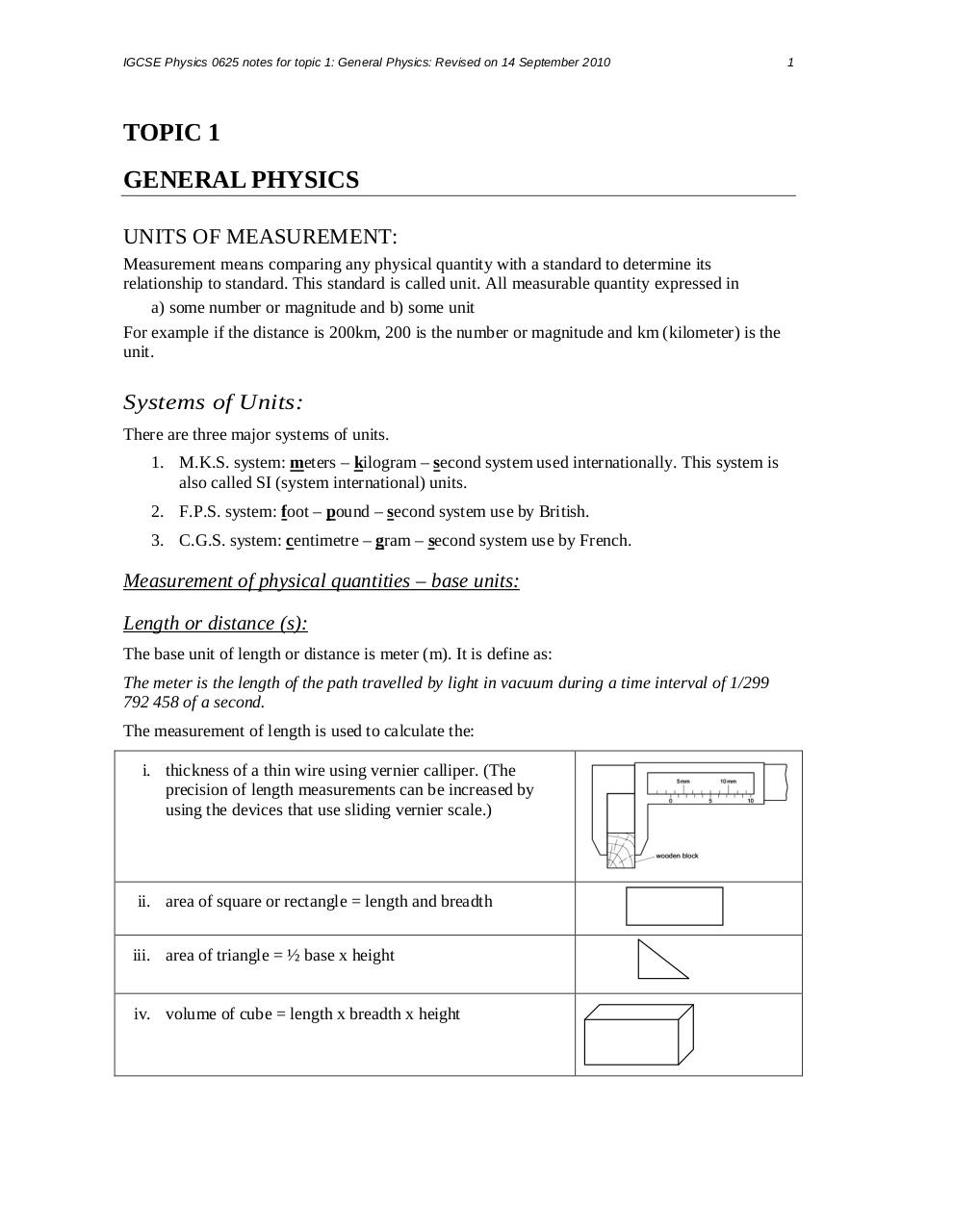# Summary of Chapter 2,3,4.pdfPage 12317

#### Text preview

IGCSE Physics 0625 notes for topic 1: General Physics: Revised on 14 September 2010

TOPIC 1
GENERAL PHYSICS
UNITS OF MEASUREMENT:
Measurement means comparing any physical quantity with a standard to determine its
relationship to standard. This standard is called unit. All measurable quantity expressed in
a) some number or magnitude and b) some unit
For example if the distance is 200km, 200 is the number or magnitude and km (kilometer) is the
unit.

Systems of Units:
There are three major systems of units.
1. M.K.S. system: meters – kilogram – second system used internationally. This system is
also called SI (system international) units.
2. F.P.S. system: foot – pound – second system use by British.
3. C.G.S. system: centimetre – gram – second system use by French.

Measurement of physical quantities – base units:
Length or distance (s):
The base unit of length or distance is meter (m). It is define as:
The meter is the length of the path travelled by light in vacuum during a time interval of 1/299
792 458 of a second.
The measurement of length is used to calculate the:
i. thickness of a thin wire using vernier calliper. (The
precision of length measurements can be increased by
using the devices that use sliding vernier scale.)

ii. area of square or rectangle = length and breadth
iii. area of triangle = ½ base x height

iv. volume of cube = length x breadth x height

1